# The value of g ( x ) , for x = 0 , x = 1 , x = 2 , x = 3 , x = 4 , x = 5 , and x = 6 .### Single Variable Calculus: Concepts...

4th Edition
James Stewart
Publisher: Cengage Learning
ISBN: 9781337687805### Single Variable Calculus: Concepts...

4th Edition
James Stewart
Publisher: Cengage Learning
ISBN: 9781337687805

#### Solutions

Chapter 5.4, Problem 2E

(a)

To determine

## The value of g(x), for x=0, x=1, x=2, x=3, x=4, x=5, and x=6.

Expert Solution

The value of g(x) is 0.

The value of g(1) is 12_

The value of g(2) is 0_.

The value of g(3) is 12_.

The value of g(4) is 0_.

The value of g(5) is 1.5_.

The value of g(6) is 4_.

### Explanation of Solution

Given information:

The equation is g(x)=0xf(t)dt.

Calculation:

Show the integral function as below:

g(x)=0xf(t)dt (1)

Here, g(x) is the area under the graph of f from a to x and f(t) is function of t.

Determine g(0) using Equation (1).

Substitute 0 for x in Equation (1).

g(0)=00f(t)dt=0

Therefore, g(0) is 0_.

Draw the graph for the calculation of g(1) as in Figure (1).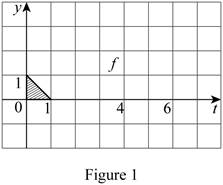Determine g(1) using Equation (1).

Substitute 1 for x in Equation (1).

g(1)=01f(t)dt (2)

Refer to Figure (1).

The area of the shaded triangle is the function of t with limits 0 to 1.

Modify Equation (2).

g(1)=12bh

Substitute 1 for b and 1 for h.

g(1)=12bh=12(1)(1)=12

Therefore, g(1) is 12_.

Draw the graph for the calculation of g(2) as in Figure (2).Determine g(2) using Equation (1).

Substitute 2 for x in Equation (1).

g(2)=02f(t)dt=01f(t)dt+12f(t)dt=g(1)+12f(t)dt (3)

Refer to Figure (2).

The area of the shaded triangle is the function of t with limits 1 to 2.

Substitute 12 for g(1) and (12bh) for 12f(t)dt in Equation (3).

g(2)=g(1)+12f(t)dt=12+12bh

Substitute 1 for b and -1 for h.

g(2)=12+12bh=12+12(1)(1)=0

Therefore, g(2) is 0_.

Draw the graph for the calculation of g(3) as in Figure (3).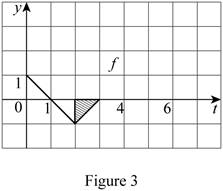Determine g(3) using Equation (1).

Substitute 3 for x in Equation (1).

g(3)=03f(t)dt=01f(t)dt+12f(t)dt+23f(t)dt=g(2)+23f(t)dt (4)

Refer to Figure 3.

The area of the shaded triangle is the function of t with limits 2 to 3.

Substitute 0 for g(2) and (12bh) for 32f(t)dt in Equation (4).

g(3)=g(2)+32f(t)dt=0+12(b)(h)=12bh

Substitute 1 for b and -1 for h.

g(3)=12bh=12(1)(1)

Therefore, g(3) is 12_.

Draw the graph for the calculation of g(4) as in Figure (4).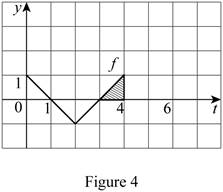Determine g(4) using Equation (1).

Substitute 4 for x in Equation (1).

g(4)=04f(t)dt=01f(t)dt+12f(t)dt+23f(t)dt+34f(t)dt=g(3)+34f(t)dt (5)

Refer to Figure 4.

The area of the shaded triangle is the function of t with limits 3 to 4.

Substitute 12 for g(3) and (12bh) for 34f(t)dt in Equation (5).

g(4)=g(3)+34f(t)dt=12+12bh

Substitute 1 for b and 1 for h.

g(4)=12+12bh=12+12(1)(1)=0

Therefore, g(4) is 0.

Draw the graph for the calculation of g(5) as in Figure (5).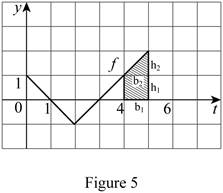Determine g(5) using Equation (1).

Substitute 5 for x in Equation (1).

g(5)=05f(t)dt=01f(t)dt+12f(t)dt+23f(t)dt+34f(t)dt+45f(t)dt=g(4)+45f(t)dt (6)

Refer to Figure 5.

The area of the shaded portion is the function of t with limits 4 to 5.

Substitute 0 for g(4) and (b1h1+12b2h2) for 45f(t)dt in Equation (6).g(5)=g(4)+45f(t)dt=0+(b1h1+12b2h2)=b1h1+12b2h2

Substitute 1 for b1, 1 for h1, 1 for b2, and 1 for h2.

g(5)=b1h1+12b2h2=(1×1)+(12×1×1)=1+12=1.5

Therefore, the g(5) is 1.5_.

Draw the graph for the calculation of g(6) as in Figure (6).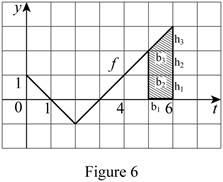Determine g(6) using Equation (1).

Substitute 6 for x in Equation (1).

g(6)=05f(t)dt=01f(t)dt+12f(t)dt+23f(t)dt+34f(t)dt+45f(t)dt+56f(t)dt=g(5)+56f(t)dt (7)

Refer to Figure 6.

The area of the shaded portion is the function of t with limits 5 to 6.

Substitute 1.5 for g(5) and (b1h1+b2h2+12b3h3) for 56f(t)dt in Equation (7).g(6)=g(5)+56f(t)dt=1.5+(b1h1+b2h2+12b3h3)

Substitute 1 for b1, 1 for h1, 1 for b2, and 1 for h2, 1 for b3, and 1 for h3.

g(6)=1.5+(b1h1+b2h2+12b3h3)=1.5+[(1×1)+(1×1)+(12×1×1)]=4

Therefore, g(6) is 4_.

(b)

To determine

### The value of g(x) at x=7.

Expert Solution

The value of g(7) is 6.2_.

### Explanation of Solution

Given information:

The equation is g(x)=0xf(t)dt.

Calculation:

Draw the graph for the calculation of g(7) as in Figure (7).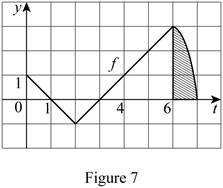Determine g(7) using Equation (1).

Substitute 7 for x in Equation (1).

g(7)=05f(t)dt=(01f(t)dt+12f(t)dt+23f(t)dt+34f(t)dt+45f(t)dt+56f(t)dt+67f(t)dt)=g(6)+67f(t)dt (8)

Refer to Figure 7.

The area of the shaded portion is the function of t with limits 6 to 7; it is bounded by a curved shape. Hence, take the approximate value from the graph

Take the approximate area of the shaded portion as 2.2

Substitute 4 for g(6) and 2.2 for 67f(t)dt in Equation (8).

g(7)=g(6)+67f(t)dt=4+2.2=6.2

Therefore, g(7) is 6.2_.

(c)

To determine

### The maximum and minimum value of g.

Expert Solution

The minimum value of g lies at x=3_ and maximum value lies at x=7_.

### Explanation of Solution

Given information:

The equation is g(x)=0xf(t)dt.

Calculation:

From the calculation in Part (A),

The minimum value of function of g is 12 at x=3.

The maximum value of function of g is 6.2 at x=7

Therefore, the minimum value of g lies at x=3_ and maximum value is lies at x=7_.

(d)

To determine

Expert Solution

### Explanation of Solution

Plot the graph for function f using the calculated values of 0, 12, 0, 12, 0, 1.5, 4, and for the functions g(0), g(1), g(2), g(3), g(4), g(5), g(6), and g(7) respectively.

Draw the graph for the function of f as in Figure (8).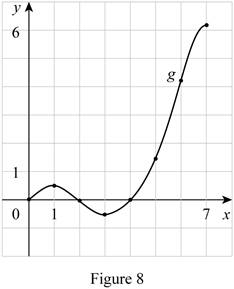### Have a homework question?

Subscribe to bartleby learn! Ask subject matter experts 30 homework questions each month. Plus, you’ll have access to millions of step-by-step textbook answers!